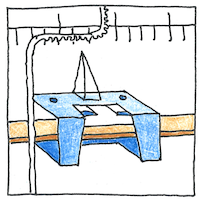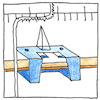# 1888 Ampere balance

## The book of science

Tom Sharp

 William Thomson, Lord Kelvin electromagnetism## Ampere balance

• To measure the ampere,
• slide a weight on a scale
• to balance the current to be measured
• coiled against the weight.

## Ampere calculation

• The strength of the current
• when the weight is balanced
• is proportional to the product
• of the square root of the weight
• and its displacement
• from its zero position.

## Balance

• Nothing is that delicately balanced
• that the result comes from a simple equation.
• The scale must be level; we must know precisely
• the dimension and winding of the coil;
• and how do we measure the mechanical rigidity
• In balancing a current against a weight,
• either current or weight might be off.
• We should apply Bayes’ theorem
• and balance the significance of what we know
• against the weight of what we don’t know.

The Kibble balance is seen as a more accurate version of the Ampere balance even though Kibble’s purpose was to measure a weight and Kelvin’s purpose was to measure a current.